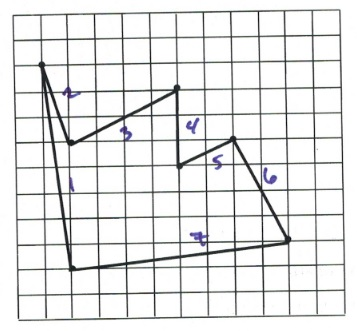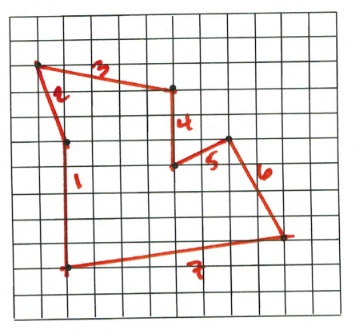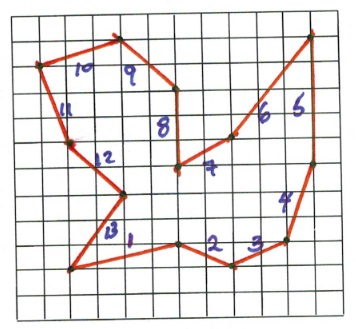Chapter 3: Graphing

# 3.8 The Newspaper Delivery Puzzle

A newspaper delivery driver wants to visit of number of specific stores in a city to drop off bundles of newspapers and return to the starting position by taking the shortest distance. Since there are a number of stores and choices of what path is best to take, what is the shortest distance you can find?

One possible path is shown below. Can you find the distance covered in this path if each square represents 1 km?$\begin{array}{rrlrl} d_1&=&\sqrt{8^2+1^2}&=&\phantom{0}8.062 \\ d_2&=&\sqrt{3^2+1^2}&=&\phantom{0}3.612 \\ d_3&=&\sqrt{4^2+2^2}&=&\phantom{0}4.472 \\ d_4&=&3&=&\phantom{0}3 \\ d_5&=&\sqrt{1^2+2^2}&=&\phantom{0}2.236 \\ d_6&=&\sqrt{4^2+2^2}&=&\phantom{0}4.472 \\ d_7&=&\sqrt{8^2+1^2}&=&\phantom{0}8.062 \\ \hline &&&&33.916 \text{ km} \end{array}$

Can you find a shorter path? How many km of driving would you save?$\begin{array}{rrlrl} d_1&=&5&=&\phantom{0}5 \\ d_2&=&\sqrt{3^2+1^2}&=&\phantom{0}3.612 \\ d_3&=&\sqrt{5^2+1^2}&=&\phantom{0}5.099 \\ d_4&=&3&=&\phantom{0}3 \\ d_5&=&\sqrt{1^2+2^2}&=&\phantom{0}2.236 \\ d_6&=&\sqrt{4^2+2^2}&=&\phantom{0}4.472 \\ d_7&=&\sqrt{8^2+1^2}&=&\phantom{0}8.062 \\ \hline &&&&31.481 \text{ km} \end{array}$

These problems can rapidly become more difficult as the number of stops are increased. An example of this is as follows. What is the shortest distance that you can find for this puzzle?$\begin{array}{lrlrl} d_1&=&\sqrt{4^2+1^2}&=&\phantom{0}4.123 \\ d_2&=&\sqrt{1^2+2^2}&=&\phantom{0}2.236 \\ d_3&=&\sqrt{1^2+2^2}&=&\phantom{0}2.236 \\ d_4&=&\sqrt{1^2+3^2}&=&\phantom{0}3.162 \\ d_5&=&5&=&\phantom{0}5 \\ d_6&=&\sqrt{3^2+4^2}&=&\phantom{0}5 \\ d_7&=&\sqrt{2^2+1^2}&=&\phantom{0}2.236 \\ d_8&=&3&=&\phantom{0}3 \\ d_9&=&\sqrt{2^2+2^2}&=&\phantom{0}2.828 \\ d_{10}&=&\sqrt{1^2+3^2}&=&\phantom{0}3.612 \\ d_{11}&=&\sqrt{1^2+3^2}&=&\phantom{0}3.612 \\ d_{12}&=&\sqrt{2^2+2^2}&=&\phantom{0}2.828 \\ d_{13}&=&\sqrt{2^2+3^2}&=&\phantom{0}3.606 \\ \hline &&&&43.929 \text{ km} \end{array}$

# Why UPS Drivers Don’t Turn Left and You Probably Shouldn’t Either

The company UPS has analyzed delivery routs thoroughly. Read this article by Graham Kendall to find out how this affects the routes their drivers take.

Why UPS drivers don’t turn left and you probably shouldn’t either

1. Kendall, Graham. Why UPS Drivers Don't Turn Right and Why You Probably Shouldn't Either. https://theconversation.com/why-ups-drivers-dont-turn-left-and-you-probably-shouldnt-either-71432